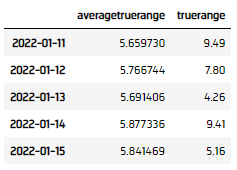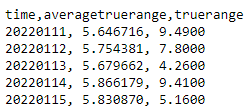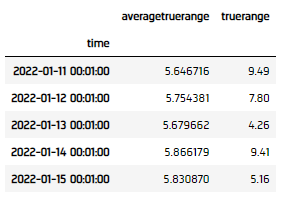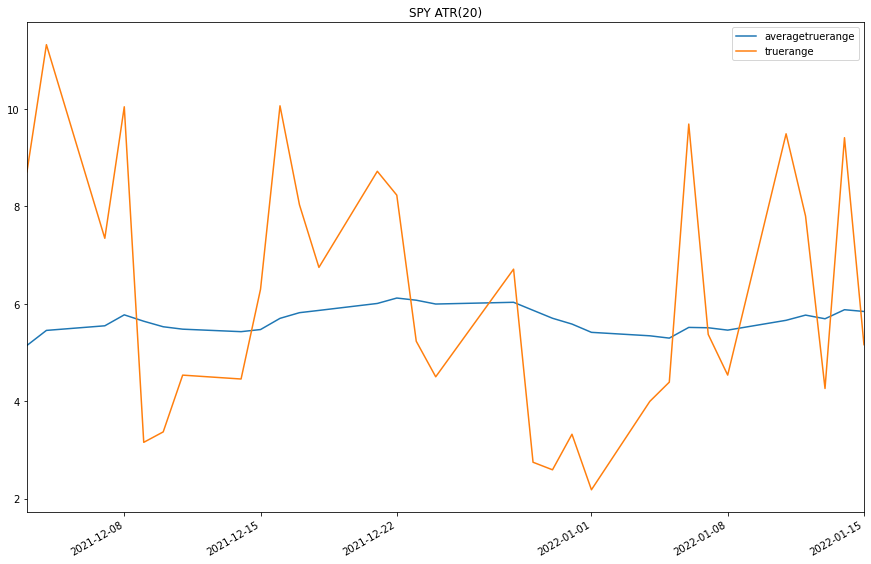# Indicators

## Bar Indicators

### Introduction

This page explains how to create, update, and visualize LEAN bar indicators.

### Create Subscriptions

You need to subscribe to some market data in order to calculate indicator values.

var qb = new QuantBook();
var symbol = qb.AddEquity("SPY").Symbol;
qb = QuantBook()
symbol = qb.AddEquity("SPY").Symbol

### Create Indicator Timeseries

You need to subscribe to some market data and create an indicator in order to calculate a timeseries of indicator values. In this example, use a 20-period AverageTrueRange indicator.

var atr = new AverageTrueRange(20);
atr = AverageTrueRange(20)

You can create the indicator timeseries with the Indicator helper method or you can manually create the timeseries.

#### Indicator Helper Method

To create an indicator timeseries with the helper method, call the Indicator method.

var atrIndicator = qb.Indicator(atr, symbol, 50, Resolution.Daily);
atr_dataframe = qb.Indicator(atr, symbol, 50, Resolution.Daily)#### Manually Create the Indicator Timeseries

Follow these steps to manually create the indicator timeseries:

1. Get some historical data.
2. var history = qb.History(symbol, 70, Resolution.Daily);
history = qb.History[TradeBar](symbol, 70, Resolution.Daily)
3. Create a RollingWindow for each attribute of the indicator to hold their values.
4. var time = new RollingWindow<DateTime>(50);
var window = new Dictionary<string, RollingWindow<decimal>>();
window["averagetruerange"] = new RollingWindow<decimal>(50);
window["truerange"] = new RollingWindow<decimal>(50);

window = {}
window['time'] = RollingWindow[DateTime](50)
window['averagetruerange'] = RollingWindow[float](50)
window["truerange"] = RollingWindow[float](50)

5. Attach a handler method to the indicator that updates the RollingWindow objects.
6. atr.Updated += (sender, updated) =>
{
var indicator = (AverageTrueRange)sender;
};
def UpdateAverageTrueRangeWindow(sender: object, updated: IndicatorDataPoint) -> None:
indicator = sender

atr.Updated += UpdateAverageTrueRangeWindow

When the indicator receives new data, the preceding handler method adds the new IndicatorDataPoint values into the respective RollingWindow.

7. Iterate through the historical market data and update the indicator.
8. foreach(var bar in history){
// Update the indicators with the whole bar.
atr.Update(bar);
}
for bar in history:
atr.Update(bar)
9. Display the data.
10. Console.WriteLine($"time,{string.Join(',', window.Select(kvp => kvp.Key))}"); foreach (var i in Enumerable.Range(0, 5).Reverse()) { var data = string.Join(", ", window.Select(kvp => Math.Round(kvp.Value[i],6))); Console.WriteLine($"{time[i]:yyyyMMdd}, {data}");
}11. Populate a DataFrame with the data in the RollingWindow objects.
12. atr_dataframe = pd.DataFrame(window).set_index('time')### Plot Indicators

Jupyter Notebooks don't currently support libraries to plot historical data, but we are working on adding the functionality. Until the functionality is added, use Python to plot bar indicators.

You need to create an indicator timeseries to plot the indicator values.

Follow these steps to plot the indicator values:

1. Call the plot method.
2. atr_indicator.plot(title="SPY ATR(20)", figsize=(15, 10))
3. Show the plots.
4. plt.show()You can also see our Videos. You can also get in touch with us via Discord.